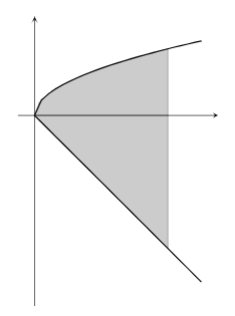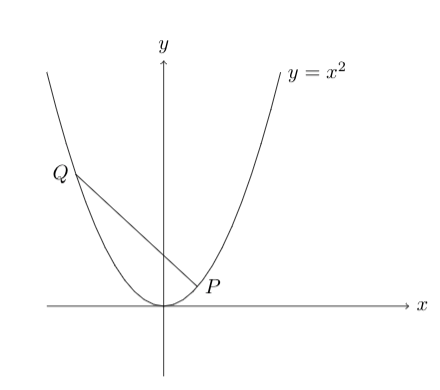# MT #5: Integral Calculus

## Part A: Short-answer type questions

Submission file: Write answers to all the ten questions on a single sheet of paper. Email a picture of your answer sheet. Name the file as PartA.jpg.

For this part, answers must be written without any explanation.

1. Suppose $$n$$ is a fixed positive integer. If $$\int_{1}^{k} x^{n-1} dx = \frac{1}{n}$$, what is the value of $$k$$?
2. Evaluate $$\displaystyle \int_{0}^{2} \frac{1}{\left( x-\frac{1}{2} \right)^2+\frac{3}{4}} dx$$.
3. Let $$f(x)$$ be a continuous real-valued function on $$[0, 1]$$ for which $\int_{0}^{1} f(x)dx = 0 \text{ and }\;\;\; \int_{0}^{1} xf(x) dx = 1$ Give an example of such a function.
4. Consider the integral $$\int_{0}^{\infty} \frac{ \sin^2 x }{\pi^2 - x^2} dx$$. Which of these statements are true?
(a) The integral does not exist as the function is not defined at $$x=\pi$$.
(b) There is a removable singularity at $$x=\pi$$ but the integral does not exist.
(c) There is a removable singularity at $$x=\pi$$ and the integral exists.
5. Find the volume of the solid obtained when the region bounded by $$y=\sqrt{2x}$$, $$y=-x$$ and the line $$x=6$$ is revolved around the x-axis.6. Let $$f(x) = \sum_{n=0}^{\infty} \frac{x2^n}{n!}$$ be a function defined in the interval $$[0,10]$$. Find the value of: $\int_{0}^{10} f(x) dx$
7. For any real number $$x$$, let $$[x]$$ denote the greatest integer $$m$$ such that $$m \leq x$$. Find the value of $$\int_{-2}^{2} [x^2 – 2]dx$$.
8. What is the area bounded by the curve $$y = \ln x$$, the x-axis and the straight line $$x=3$$?
9. Suppose $$f(x)$$ is a double differentiable function with $$f^{\prime}(0) = 1$$ and $$f^{\prime}(1) = 2$$. What is the value of the integral below? If it possible for the integral to take multiple values, write Indeterminate. $\int_{0}^{1} f^\prime(x) f^{\prime\prime}(x) dx$
10. Compute the limit: $\lim_{n\rightarrow\infty} n^3 \int_{0}^{1/n} x^{100x+2} dx$

## Part B: Subjective questions

Submission files: Each question in this part must be answered on a page of its own. Name the files as B1.jpg, B2.jpg, etc. In case you have multiple files for the same question, say B4, name the corresponding files as B4-1.jpg, B4-2.jpg, etc.

Clearly explain your entire reasoning. No credit will be given without reasoning. Partial solutions may get partial credit.

B1. Let $$f:[0,1] \rightarrow \mathbb{R}^+$$ be a continous function such that $$\int_{0}^{1} f(t) dt = \frac{1}{3}$$. Show that there exists $$c\in(0,1)$$ such that $$\int_{0}^{c} f(t) dt = c-\frac{1}{2}$$.

B2. Let $$f(x)$$ be a real-valued function defined on the interval $$[1,\infty)$$. The following equations hold: \begin{align*} f(1) &= 1 \\ f^{\prime}(x) &= \frac{1}{x^2+f(x)^2} \end{align*}

Prove that $$\lim_{x\rightarrow \infty} f(x) < 1 + \pi/4$$.

B3. Consider the parabola given by $$y = x^2$$. The normal is constructed at a point $$P$$ and meets the parabola again in $$Q$$. Determine the coordinate of $$P$$ for which the arc length along the parabola between $$P$$ and $$Q$$ is minimized.B4. Let $$f(x)$$ be a continous function defined on the interval $$I=[0,1]$$ with the property $yf(x) + xf(y) \leq 1$ for $$x,y$$ in $$I$$.
(a) Prove that: $\int_{0}^{1} f(x) dx \leq \frac{\pi}{4}$ (b) Find a function $$f(x)$$ for which equality holds in problem (a).

B5. Suppose $$\displaystyle I_{n}= \int_{0}^{2} (2x-x^2)^{n}dx$$. Prove that $$\displaystyle \lim_{n\rightarrow \infty} I_{n} = 0$$.

B6. Let $$\displaystyle f(x) = \frac{x}{1+x^5\sin^2x}$$. Prove that $$\displaystyle \int_0^\infty f(x)\; dx$$ is bounded.